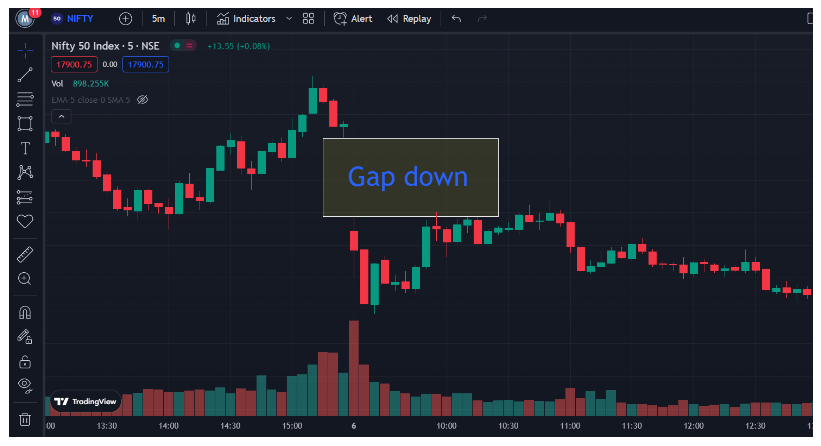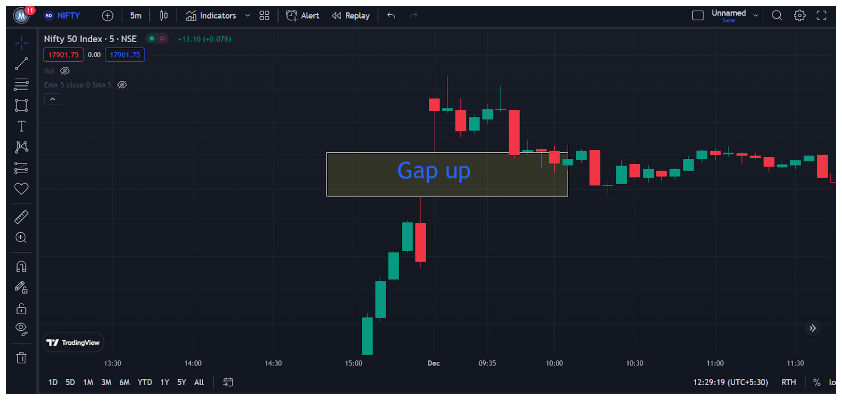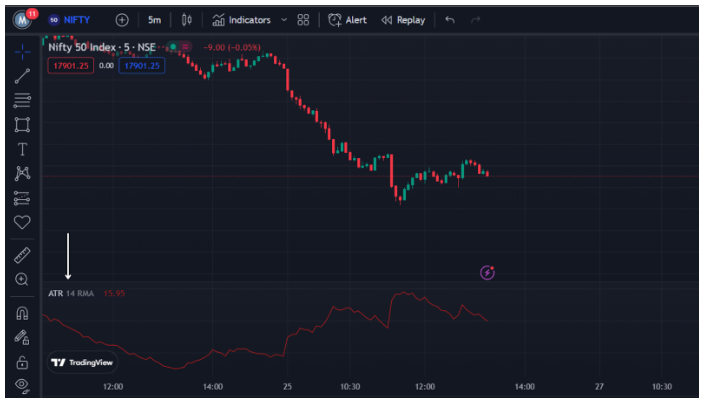# Understanding ATR indicator

Making money from the markets is not a cakewalk. Something which makes it difficult along with other factors is Volatility. ATR indicators help us to measure Volatility.

Volatility is frequently used to describe the degree of risk or uncertainty associated with the extent of variations in a security’s value. A security’s price can fluctuate in any direction because of market forces i.e. Demand and Supply. High volatility means the price of the security can fluctuate sharply over a short period of time. Lower volatility refers to stable movement rather than dramatic fluctuations.

Now that we have understood what Volatility is, let’s learn what is ATR indicator and learn how to measure it.

The Average True Range Indicator, or ATR, is a volatility indicator. When there is basically no trading between two sessions, an ATR trader can comprehend the absence of volatility. This absence of volatility leads to gaps while trading. Gaps that every amateur trader/investors know about are a gap up and a gap down. Refer to the pictures below for examples.Security has a gap down when its opening price is lower than its prior day’s closing price.When security begins at a higher price than it closed on the previous day, this is known as a gap-up.

The ATR indicator calculates the true range of the security and its average and helps the trader to bridge gaps and organize trades more efficiently. The price or trend direction are two things that the ATR won’t reveal to you. Simply put, that’s not what the indicator was intended to do.

The ATR was created by J. Welles Wilder for the commodities market, but it can also be used for stocks, futures, options, and currencies as every other technical analysis tool.

## How to apply ATR on charts?

Just like every other indicator, first, click on the indicators section of your charting platform and search for ATR or Average True Range. A single click on the indicator and you will have ATR in action. Refer to the picture below for a better understanding.Now that we have understood the meaning of the indicator, let’s learn the practical application of the ATR indicator.

When the ATR value is above 15 it is considered a highly volatile stock, one should ideally put a larger Stop Loss as the fluctuations might falsely hit a small one. When the ATR value is below 15, one can consider that as a stable stock and put a small and reasonable stop loss and ride the whole trend. When riding the trend, don’t forget to place a trailing stop loss. This is for 5 min Time frame only.

## How to calculate the ATR Indicator value?

There are 3 major components required to calculate the ATR indicator value.

1. The number of periods (n) which, in most cases, is 14. This comes already set by the charting platforms.
2. We are free to select a timeframe based on your analysis, but keep in mind that a longer timeframe can provide you with more accurate trade signals.
3. The second is obtaining true ranges from one of the three approaches.
1. Current High – Current Low
2. Current High – Previous Close
3. Current Low – Previous Close

The highest TR value among them will be used to determine the ATR.

After doing this we will get done with the first set of true ranges, but the ATR is an average of true ranges over a period of time. For calculating the value of 2nd set onward, we can use the formula given below.

ATR Formula Calculation = {[First ATR x (n-1)] + Current TR} / n

This calculation will give you values that are solely subjectively categorised as high or low. The reason is, this value indicates volatility not direction. Higher the value, the higher the volatility. Lower the value, lower the volatility.

## How to determine Stop Loss with the ATR indicator?

One can determine the Stop Loss by simply doubling the value of the ATR indicator at that value. When taking a bullish trade, 2x of ATR is below the entry price, and when taking a bearish trade, 2x of ATR is above the entry price.

Let’s take an example to understand it better. A share was trading at 1000 Rupees with an ATR indicator value of 20 at that time. The Stop Loss for a bullish trade will be 960 and for a bearish trade, it will be 1040.

## Final words

Now that you have understood the meaning and use of the ATR indicator, open Demat Account with Angel One and start building wealth with this powerful indicator.